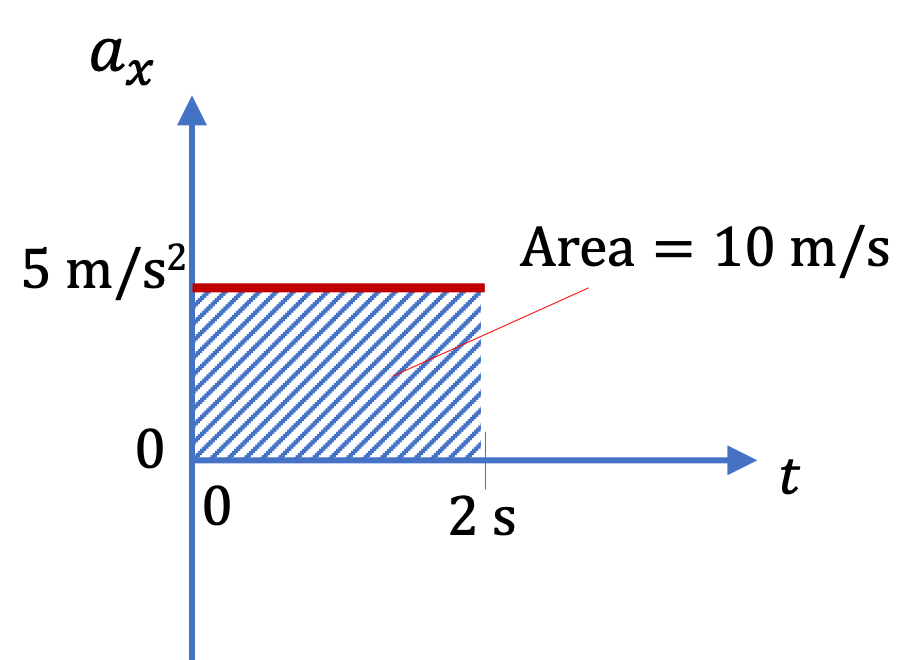## Section4.6Velocity from Acceleration

Often acceleration at each instant of an interval is known, and we want know the change in velocity during that interval. Just like getting change in position from velocity, this is also an integration or area-under-the-curve problem.

### Subsection4.6.1Area under the Acceleration Plot

Say, you have a constant acceleration in the $x$ direction of $a_{x} = 5\text{ m/s}^2\text{.}$ How much will $v_x$ change in $\Delta t = 2\text{ s}$ interval? Analytically, you will do the following.

\begin{equation*} \Delta v_x = a_x \Delta t = 5\text{ m/s}^2 \times 2\text{ s} = 10 \text{ m/s}. \end{equation*}

We can understand this in a plot of $a_x$ vs $t$ as well. Since $a_x$ is constant, the product $a_x \Delta t$ is just the area under the plot of $a_x$ vs $t\text{.}$ This gives us following general rule, which also applies to varying $a_x\text{.}$

\begin{align*} \Delta v_x \amp = \text{ Area under }a_x\text{ vs } t,\\ \amp = 5\text{ m/s}^2 \times 2\text{ s} = 10 \text{ m/s}. \end{align*}If $a_x$ is negative, then this would be area above the plot since $a_x=0$ line would be above the plot. In this case area will be negative.

The procedure clearly extends to $y$ and $z$ components as well. Once we know the change along different directions, we can combine the components to obtain the magnitude and direction of $\Delta \vec v\text{,}$ as usual for vector quantities.

Figure 4.6.2 below shows acceleration component $a_x$ of a ball at different times. The $x$ component of the velocity of the ball at $t = 0$ was $v_x = 3 \text{ m/s}\text{.}$ Find its $v_x$ at the following instants (a) $t = 5\text{ sec}\text{,}$ and (b) $t = 10\text{ sec}\text{.}$

Hint

Use area to get change and then add change to intial value.

(a) $10.5\text{ m/s}\text{,}$ (b) $0.5 \text{ m/s}\text{.}$

Solution 1 (a)

(a) We will find the change in $v_x$ during $t=0$ to $t=5\text{ sec}\text{.}$ Then we will get $v_x$ at $t=5\text{ sec}$ by adding this change to the $v_x$ at $t=0\text{.}$

\begin{equation*} v_x(\text{at } t=5\text{ sec}) = v_x(\text{at } t=0) + \Delta v_x, \end{equation*}

where

\begin{equation*} \Delta v_x = \text{Area under the curve of }a_x \text{ between }t=0\text{ and }t=5. \end{equation*}

From the plot we find this to give

\begin{equation*} \Delta v_x = \dfrac{1}{2}\times 5\text{ s} \times 3 \text{ m/s}^2 = 7.5\text{ m/s}. \end{equation*}

Therefore,

\begin{equation*} v_x(\text{at } t=5\text{ sec}) = 3\text{ m/s} + 7.5\text{ m/s} = 10.5\text{ m/s}. \end{equation*}
Solution 2 (b)

(b) We can similarly add to this the area between $t=5\text{ sec}$ and $t=10\text{ s}$ to obtain $v_x$ at $10\text{ s}\text{.}$ Now the $\Delta v_x$ is

\begin{equation*} \Delta v_x = 5\text{ s}\times (-2\text{ m/s}^2) = -10\text{ m/s}. \end{equation*}

Therefore, $v_x$ at $t=10\text{ s}$ is

\begin{equation*} v_x(\text{at } t=10\text{ s}) = 10.5\text{ m/s} + (-10\text{ m/s}) = 0.5 \text{ m/s}. \end{equation*}

A box slides on a floor in a straight path such that the magnitude of the acceleration is not constant in time. The data is collected with respect to a Cartesian axis system in which only the $a_x$ is non-zero. The $x$-component of the acceleration is shown in Figure 4.6.4. As the figure shows, the direction of the acceleration is always pointed towards positive $x$-axis, but the magnitude varies with time.

(a) Find the velocity of the box at $t = 4$ sec if it starts out at rest at $t = 0\text{.}$

(b) Find the velocity of the box at $t = 10$ sec.

Hint

Area under the curve will give $\Delta v_x\text{.}$

(a) $6\text{ m/s}\text{,}$ (b) $15\text{ m/s}\text{.}$

Solution 1 (a)

(a) We work with the duration $t = 0$ to $t = 4\text{ sec}\text{.}$ The area under the curve is just area of a triangle of base $4\text{ sec}$ and height $3\text{ m/s}^2\text{.}$ Therefore,

\begin{equation*} v_f = v_i + \Delta v = 0 + \dfrac{1}{2}\times 4\text{ s}\times 3\text{ m/s}^2 = 6\text{ m/s}. \end{equation*}
Solution 2 (b)

(b) We work with the duration $t = 0$ to $t = 10\text{ sec}\text{.}$ The area under the curve is just area of a triangle of base $10\text{ sec}$ and height $3\text{ m/s}^2\text{.}$ Therefore,

\begin{equation*} v_f = v_i + \Delta v = 0 + \dfrac{1}{2}\times 10\text{ s}\times 3\text{ m/s}^2 = 15\text{ m/s}. \end{equation*}

A hockey puck is shot on a surface that has different roughness at different places. As a result its acceleration varies from place to place. By placing the $x$-axis on the line of motion of the puck, we cast the acceleration vector in terms of its $x$-component which can be plotted with time. Note the acceleration vector cannot be plotted, since they are not ordinary functions; only the components can be plotted. The resulting $x$-component of the acceleration is shown Figure 4.6.6. The $y$ and $z$-components of the acceleration are zero. The puck has a velocity at $t = 5$ sec of $2$ m/s towards the positive $x$ axis. Find the initial velocity of the puck at $t = 0\text{.}$

Hint

Area under the curve will give $\Delta v_x\text{.}$

$21.75\text{ m/s}\text{.}$

Solution

The areas we need are shaded in Figure 4.6.7. The area will be negative here.

I found the area to be

\begin{align*} \Delta v \amp = -4\times 3.5 + (-1 )\times 1.5+ \dfrac{(-6+4) \times 2}{2} + \dfrac{(-4+1)\times 1.5}{2} \\ \amp = -19.75\text{ m/s}. \end{align*}

Therefore,

\begin{equation*} v_f - v_i = \Delta v, \end{equation*}

gives

\begin{equation*} v_i = v_f - \Delta v = 2\text{ m/s} - ( -19.75\text{ m/s}) = 21.75\text{ m/s}. \end{equation*}

### Subsection4.6.2(Calculus) Integrating the Acceleration

Along the $x$ component of the motion the relation between velocity and acceleration will be

\begin{equation} \dfrac{dv_x}{dt} = a_x \Longleftrightarrow v_{f,x} - v_{i,x} = \int_{t_i}^{t_f}\, a_x(t)\, dt.\label{eq-velocity-from-acceleration-x-fundamental}\tag{4.6.1} \end{equation}

Applying this similarly to $y$ and $z$ motions gives us changes in $v_y$ and $v_z \text{.}$

The integral in the $x$ motion can be evaluated either by integrating an analytic expression of $a_x$ or by the “area under the curve method” of evaluating integrals, discussed in the displacement from velocity section.